Prealgebra

# 11.1Use the Rectangular Coordinate System

Prealgebra11.1 Use the Rectangular Coordinate System

### Learning Objectives

By the end of this section, you will be able to:
• Plot points on a rectangular coordinate system
• Identify points on a graph
• Verify solutions to an equation in two variables
• Complete a table of solutions to a linear equation
• Find solutions to linear equations in two variables

### Be Prepared 11.1

Before you get started, take this readiness quiz.

1. Evaluate: $x+3x+3$ when $x=−1.x=−1.$
If you missed this problem, review Example 3.23.
2. Evaluate: $2x−5y2x−5y$ when $x=3,y=−2.x=3,y=−2.$
If you missed this problem, review Example 3.56.
3. Solve for $y:40−4y=20.y:40−4y=20.$
If you missed this problem, review Example 8.20.

### Plot Points on a Rectangular Coordinate System

Many maps, such as the Campus Map shown in Figure 11.2, use a grid system to identify locations. Do you see the numbers $1,2,3,1,2,3,$ and $44$ across the top and bottom of the map and the letters A, B, C, and D along the sides? Every location on the map can be identified by a number and a letter.

For example, the Student Center is in section 2B. It is located in the grid section above the number $22$ and next to the letter B. In which grid section is the Stadium? The Stadium is in section 4D.

Figure 11.2

### Example 11.1

Use the map in Figure 11.2.

1. Find the grid section of the Residence Halls.
2. What is located in grid section 4C?

### Try It 11.1

Use the map in Figure 11.2.

1. Find the grid section of Taylor Hall.
2. What is located in section 3B?

### Try It 11.2

Use the map in Figure 11.2.

1. Find the grid section of the Parking Garage.
2. What is located in section 2C?

Just as maps use a grid system to identify locations, a grid system is used in algebra to show a relationship between two variables in a rectangular coordinate system. To create a rectangular coordinate system, start with a horizontal number line. Show both positive and negative numbers as you did before, using a convenient scale unit. This horizontal number line is called the x-axis.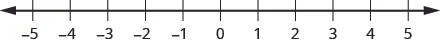Now, make a vertical number line passing through the $x-axisx-axis$ at $0.0.$ Put the positive numbers above $00$ and the negative numbers below $0.0.$ See Figure 11.3. This vertical line is called the y-axis.

Vertical grid lines pass through the integers marked on the $x-axis.x-axis.$ Horizontal grid lines pass through the integers marked on the $y-axis.y-axis.$ The resulting grid is the rectangular coordinate system.

The rectangular coordinate system is also called the $x-yx-y$ plane, the coordinate plane, or the Cartesian coordinate system (since it was developed by a mathematician named René Descartes.)

Figure 11.3 The rectangular coordinate system.

The $x-axisx-axis$ and the $y-axisy-axis$ form the rectangular coordinate system. These axes divide a plane into four areas, called quadrants. The quadrants are identified by Roman numerals, beginning on the upper right and proceeding counterclockwise. See Figure 11.4.

Figure 11.4 The four quadrants of the rectangular coordinate system

In the rectangular coordinate system, every point is represented by an ordered pair. The first number in the ordered pair is the x-coordinate of the point, and the second number is the y-coordinate of the point.

### Ordered Pair

An ordered pair, $(x,y)(x,y)$ gives the coordinates of a point in a rectangular coordinate system.

$The first number is thex-coordinate.The second number is they-coordinate.The first number is thex-coordinate.The second number is they-coordinate.$So how do the coordinates of a point help you locate a point on the $x-yx-y$ plane?

Let’s try locating the point $(2,5)(2,5)$. In this ordered pair, the $xx$-coordinate is $22$ and the $yy$-coordinate is $55$.

We start by locating the $xx$ value, $2,2,$ on the $x-axis.x-axis.$ Then we lightly sketch a vertical line through $x=2,x=2,$ as shown in Figure 11.5.

Figure 11.5

Now we locate the $yy$ value, $5,5,$ on the $yy$-axis and sketch a horizontal line through $y=5y=5$. The point where these two lines meet is the point with coordinates $(2,5).(2,5).$ We plot the point there, as shown in Figure 11.6.

Figure 11.6

### Example 11.2

Plot $(1,3)(1,3)$ and $(3,1)(3,1)$ in the same rectangular coordinate system.

### Try It 11.3

Plot each point on the same rectangular coordinate system: $(2,5),(5,2).(2,5),(5,2).$

### Try It 11.4

Plot each point on the same rectangular coordinate system: $(4,2),(2,4).(4,2),(2,4).$

### Example 11.3

Plot each point in the rectangular coordinate system and identify the quadrant in which the point is located:

1. $(−1,3)(−1,3)$
2. $(−3,−4)(−3,−4)$
3. $(2,−3)(2,−3)$
4. $(3,52)(3,52)$

### Try It 11.5

Plot each point on a rectangular coordinate system and identify the quadrant in which the point is located:

1. $(−2,1)(−2,1)$
2. $(−3,−1)(−3,−1)$
3. $(4,−4)(4,−4)$
4. $(−4,32)(−4,32)$

### Try It 11.6

Plot each point on a rectangular coordinate system and identify the quadrant in which the point is located

1. $(−4,1)(−4,1)$
2. $(−2,3)(−2,3)$
3. $(2,−5)(2,−5)$
4. $(−3,52)(−3,52)$

How do the signs affect the location of the points?

### Example 11.4

Plot each point:

1. $(−5,2)(−5,2)$
2. $(−5,−2)(−5,−2)$
3. $(5,2)(5,2)$
4. $(5,−2)(5,−2)$

### Try It 11.7

Plot each point:

1. $(4,−3)(4,−3)$
2. $(4,3)(4,3)$
3. $(−4,−3)(−4,−3)$
4. $(−4,3)(−4,3)$

### Try It 11.8

Plot each point:

1. $(−1,4)(−1,4)$
2. $(1,4)(1,4)$
3. $(1,−4)(1,−4)$
4. $(−1,−4)(−1,−4)$

You may have noticed some patterns as you graphed the points in the two previous examples.

For each point in Quadrant IV, what do you notice about the signs of the coordinates?

Can you tell just by looking at the coordinates in which quadrant the point (−2, 5) is located? In which quadrant is (2, −5) located?

We can summarize sign patterns of the quadrants as follows. Also see Figure 11.7.

(x,y) (x,y) (x,y) (x,y)
(+,+) (−,+) (−,−) (+,−)
Table 11.1
Figure 11.7

What if one coordinate is zero? Where is the point $(0,4)(0,4)$ located? Where is the point $(−2,0)(−2,0)$ located? The point $(0,4)(0,4)$ is on the y-axis and the point $(−2,0)(−2,0)$ is on the x-axis.

### Points on the Axes

Points with a $y-coordinatey-coordinate$ equal to $00$ are on the $x-axis,x-axis,$ and have coordinates $(a,0).(a,0).$

Points with an $x-coordinatex-coordinate$ equal to $00$ are on the $y-axis,y-axis,$ and have coordinates $(0,b).(0,b).$

What is the ordered pair of the point where the axes cross? At that point both coordinates are zero, so its ordered pair is $(0,0)(0,0)$. The point has a special name. It is called the origin.

### The Origin

The point $(0,0)(0,0)$ is called the origin. It is the point where the x-axis and y-axis intersect.

### Example 11.5

Plot each point on a coordinate grid:

1. $(0,5)(0,5)$
2. $(4,0)(4,0)$
3. $(−3,0)(−3,0)$
4. $(0,0)(0,0)$
5. $(0,−1)(0,−1)$

### Try It 11.9

Plot each point on a coordinate grid:

1. $(4,0)(4,0)$
2. $(−2,0)(−2,0)$
3. $(0,0)(0,0)$
4. $(0,2)(0,2)$
5. $(0,−3)(0,−3)$

### Try It 11.10

Plot each point on a coordinate grid:

1. $(−5,0)(−5,0)$
2. $(3,0)(3,0)$
3. $(0,0)(0,0)$
4. $(0,−1)(0,−1)$
5. $(0,4)(0,4)$

### Identify Points on a Graph

In algebra, being able to identify the coordinates of a point shown on a graph is just as important as being able to plot points. To identify the x-coordinate of a point on a graph, read the number on the x-axis directly above or below the point. To identify the y-coordinate of a point, read the number on the y-axis directly to the left or right of the point. Remember, to write the ordered pair using the correct order $(x,y).(x,y).$

### Example 11.6

Name the ordered pair of each point shown: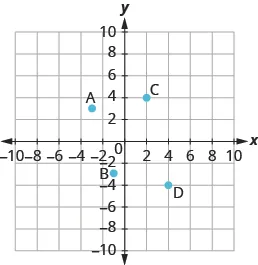### Try It 11.11

Name the ordered pair of each point shown: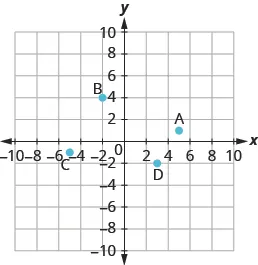### Try It 11.12

Name the ordered pair of each point shown: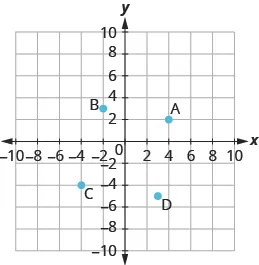### Example 11.7

Name the ordered pair of each point shown: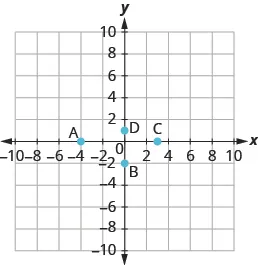### Try It 11.13

Name the ordered pair of each point shown:### Try It 11.14

Name the ordered pair of each point shown: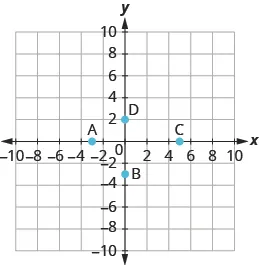### Verify Solutions to an Equation in Two Variables

All the equations we solved so far have been equations with one variable. In almost every case, when we solved the equation we got exactly one solution. The process of solving an equation ended with a statement such as $x=4.x=4.$ Then we checked the solution by substituting back into the equation.

Here’s an example of a linear equation in one variable, and its one solution.

$3x+5=173x=12x=43x+5=173x=12x=4$

But equations can have more than one variable. Equations with two variables can be written in the general form $Ax+By=C.Ax+By=C.$ An equation of this form is called a linear equation in two variables.

### Linear Equation

An equation of the form $Ax+By=C,Ax+By=C,$ where $AandBAandB$ are not both zero, is called a linear equation in two variables.

Notice that the word “line” is in linear.

Here is an example of a linear equation in two variables, $xx$ and $y:y:$Is $y=−5x+1y=−5x+1$ a linear equation? It does not appear to be in the form $Ax+By=C.Ax+By=C.$ But we could rewrite it in this form.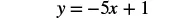Add $5x5x$ to both sides.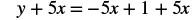Simplify.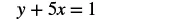Use the Commutative Property to put it in $Ax+By=C.Ax+By=C.$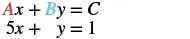By rewriting $y=−5x+1y=−5x+1$ as $5x+y=1,5x+y=1,$ we can see that it is a linear equation in two variables because it can be written in the form $Ax+By=C.Ax+By=C.$

Linear equations in two variables have infinitely many solutions. For every number that is substituted for $x,x,$ there is a corresponding $yy$ value. This pair of values is a solution to the linear equation and is represented by the ordered pair $(x,y).(x,y).$ When we substitute these values of $xx$ and $yy$ into the equation, the result is a true statement because the value on the left side is equal to the value on the right side.

### Solution to a Linear Equation in Two Variables

An ordered pair $(x,y)(x,y)$ is a solution to the linear equation $Ax+By=C,Ax+By=C,$ if the equation is a true statement when the $x-x-$ and $y-valuesy-values$ of the ordered pair are substituted into the equation.

### Example 11.8

Determine which ordered pairs are solutions of the equation $x+4y=8:x+4y=8:$

1. $(0,2)(0,2)$
2. $(2,−4)(2,−4)$
3. $(−4,3)(−4,3)$

### Try It 11.15

Determine which ordered pairs are solutions to the given equation: $2x+3y=62x+3y=6$

1. $(3,0)(3,0)$
2. $(2,0)(2,0)$
3. $(6,−2)(6,−2)$

### Try It 11.16

Determine which ordered pairs are solutions to the given equation: $4x−y=84x−y=8$

1. $(0,8)(0,8)$
2. $(2,0)(2,0)$
3. $(1,−4)(1,−4)$

### Example 11.9

Determine which ordered pairs are solutions of the equation. $y=5x−1:y=5x−1:$

1. $(0,−1)(0,−1)$
2. $(1,4)(1,4)$
3. $(−2,−7)(−2,−7)$

### Try It 11.17

Determine which ordered pairs are solutions of the given equation: $y=4x−3y=4x−3$

1. $(0,3)(0,3)$
2. $(1,1)(1,1)$
3. $(1,1)(1,1)$

### Try It 11.18

Determine which ordered pairs are solutions of the given equation: $y=−2x+6y=−2x+6$

1. $(0,6)(0,6)$
2. $(1,4)(1,4)$
3. $(−2,−2)(−2,−2)$

### Complete a Table of Solutions to a Linear Equation

In the previous examples, we substituted the $x- andy-valuesx- andy-values$ of a given ordered pair to determine whether or not it was a solution to a linear equation. But how do we find the ordered pairs if they are not given? One way is to choose a value for $xx$ and then solve the equation for $y.y.$ Or, choose a value for $yy$ and then solve for $x.x.$

We’ll start by looking at the solutions to the equation $y=5x−1y=5x−1$ we found in Example 11.9. We can summarize this information in a table of solutions.

$y=5x−1y=5x−1$
$xx$ $yy$ $(x,y)(x,y)$
$00$ $−1−1$ $(0,−1)(0,−1)$
$11$ $44$ $(1,4)(1,4)$

To find a third solution, we’ll let $x=2x=2$ and solve for $y.y.$

 $y=5x−1y=5x−1$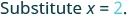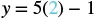Multiply. $y=10−1y=10−1$ Simplify. $y=9y=9$

The ordered pair is a solution to $y=5x-1y=5x-1$. We will add it to the table.

$y=5x−1y=5x−1$
$xx$ $yy$ $(x,y)(x,y)$
$00$ $−1−1$ $(0,−1)(0,−1)$
$11$ $44$ $(1,4)(1,4)$
$22$ $99$ $(2,9)(2,9)$

We can find more solutions to the equation by substituting any value of $xx$ or any value of $yy$ and solving the resulting equation to get another ordered pair that is a solution. There are an infinite number of solutions for this equation.

### Example 11.10

Complete the table to find three solutions to the equation $y=4x−2:y=4x−2:$

$y=4x−2y=4x−2$
$xx$ $yy$ $(x,y)(x,y)$
$00$
$−1−1$
$22$

### Try It 11.19

Complete the table to find three solutions to the equation: $y=3x−1.y=3x−1.$

$y=3x−1y=3x−1$
$xx$ $yy$ $(x,y)(x,y)$
$00$
$−1−1$
$22$

### Try It 11.20

Complete the table to find three solutions to the equation: $y=6x+1y=6x+1$

$y=6x+1y=6x+1$
$xx$ $yy$ $(x,y)(x,y)$
$00$
$11$
$−2−2$

### Example 11.11

Complete the table to find three solutions to the equation $5x−4y=20:5x−4y=20:$

$5x−4y=205x−4y=20$
$xx$ $yy$ $(x,y)(x,y)$
$00$
$00$
$55$

### Try It 11.21

Complete the table to find three solutions to the equation: $2x−5y=20.2x−5y=20.$

$2x−5y=202x−5y=20$
$xx$ $yy$ $(x,y)(x,y)$
$00$
$00$
$−5−5$

### Try It 11.22

Complete the table to find three solutions to the equation: $3x−4y=12.3x−4y=12.$

$3x−4y=123x−4y=12$
$xx$ $yy$ $(x,y)(x,y)$
$00$
$00$
$−4−4$

### Find Solutions to Linear Equations in Two Variables

To find a solution to a linear equation, we can choose any number we want to substitute into the equation for either $xx$ or $y.y.$ We could choose $1,100,1,000,1,100,1,000,$ or any other value we want. But it’s a good idea to choose a number that’s easy to work with. We’ll usually choose $00$ as one of our values.

### Example 11.12

Find a solution to the equation $3x+2y=6.3x+2y=6.$

### Try It 11.23

Find a solution to the equation: $4x+3y=12.4x+3y=12.$

### Try It 11.24

Find a solution to the equation: $2x+4y=8.2x+4y=8.$

We said that linear equations in two variables have infinitely many solutions, and we’ve just found one of them. Let’s find some other solutions to the equation $3x+2y=6.3x+2y=6.$

### Example 11.13

Find three more solutions to the equation $3x+2y=6.3x+2y=6.$

### Try It 11.25

Find three solutions to the equation: $2x+3y=6.2x+3y=6.$

### Try It 11.26

Find three solutions to the equation: $4x+2y=8.4x+2y=8.$

Let’s find some solutions to another equation now.

### Example 11.14

Find three solutions to the equation $x−4y=8.x−4y=8.$

### Try It 11.27

Find three solutions to the equation: $4x+y=8.4x+y=8.$

### Try It 11.28

Find three solutions to the equation: $x+5y=10.x+5y=10.$

### Section 11.1 Exercises

#### Practice Makes Perfect

Plot Points on a Rectangular Coordinate System

In the following exercises, plot each point on a coordinate grid.

1.

$( 3 , 2 ) ( 3 , 2 )$

2.

$( 4 , 1 ) ( 4 , 1 )$

3.

$( 1 , 5 ) ( 1 , 5 )$

4.

$( 3 , 4 ) ( 3 , 4 )$

5.

$( 4 , 1 ) , ( 1 , 4 ) ( 4 , 1 ) , ( 1 , 4 )$

6.

$( 3 , 2 ) , ( 2 , 3 ) ( 3 , 2 ) , ( 2 , 3 )$

7.

$( 3 , 4 ) , ( 4 , 3 ) ( 3 , 4 ) , ( 4 , 3 )$

In the following exercises, plot each point on a coordinate grid and identify the quadrant in which the point is located.

8.
1. $( −4 , 2 ) ( −4 , 2 )$
2. $( −1 , −2 ) ( −1 , −2 )$
3. $( 3 , −5 ) ( 3 , −5 )$
4. $( 2 , 5 2 ) ( 2 , 5 2 )$
9.
1. $( −2 , −3 ) ( −2 , −3 )$
2. $( 3 , −3 ) ( 3 , −3 )$
3. $( −4 , 1 ) ( −4 , 1 )$
4. $( 1 , 3 2 ) ( 1 , 3 2 )$
10.
1. $( −1 , 1 ) ( −1 , 1 )$
2. $( −2 , −1 ) ( −2 , −1 )$
3. $( 1 , −4 ) ( 1 , −4 )$
4. $( 3 , 7 2 ) ( 3 , 7 2 )$
11.
1. $( 3 , -2 ) ( 3 , -2 )$
2. $( −3 , 2 ) ( −3 , 2 )$
3. $( -3 , −2 ) ( -3 , −2 )$
4. $( 3 , 2 ) ( 3 , 2 )$
12.
1. $( 4 , -1 ) ( 4 , -1 )$
2. $( −4 , 1 ) ( −4 , 1 )$
3. $( -4 , -1 ) ( -4 , -1 )$
4. $( 4 , 1 ) ( 4 , 1 )$
13.
1. $( −2 , 0 ) ( −2 , 0 )$
2. $( −3 , 0 ) ( −3 , 0 )$
3. $( 0 , 4 ) ( 0 , 4 )$
4. $( 0 , 2 ) ( 0 , 2 )$

Identify Points on a Graph

In the following exercises, name the ordered pair of each point shown.

14.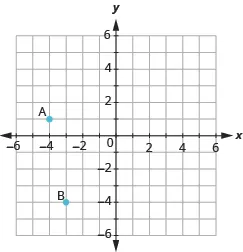15.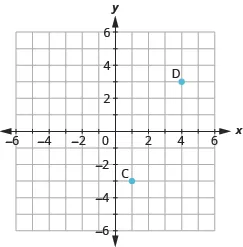16.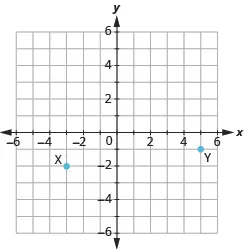17.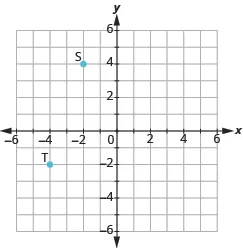18.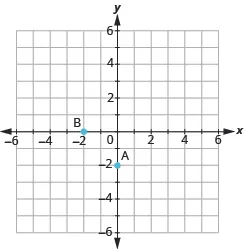19.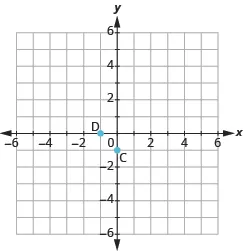20.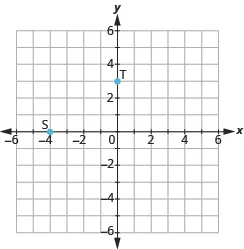Verify Solutions to an Equation in Two Variables

In the following exercises, determine which ordered pairs are solutions to the given equation.

21.

$2 x + y = 6 2 x + y = 6$

1. $( 1 , 4 ) ( 1 , 4 )$
2. $( 3 , 0 ) ( 3 , 0 )$
3. $( 2 , 3 ) ( 2 , 3 )$
22.

$x + 3 y = 9 x + 3 y = 9$

1. $( 0 , 3 ) ( 0 , 3 )$
2. $( 6 , 1 ) ( 6 , 1 )$
3. $( -3 , -3 ) ( -3 , -3 )$
23.

$4 x − 2 y = 8 4 x − 2 y = 8$

1. $( 3 , 2 ) ( 3 , 2 )$
2. $( 1 , 4 ) ( 1 , 4 )$
3. $( 0 , -4 ) ( 0 , -4 )$
24.

$3 x − 2 y = 12 3 x − 2 y = 12$

1. $( 4 , 0 ) ( 4 , 0 )$
2. $( 2 , -3 ) ( 2 , -3 )$
3. $( 1 , 6 ) ( 1 , 6 )$
25.

$y = 4 x + 3 y = 4 x + 3$

1. $( 4 , 3 ) ( 4 , 3 )$
2. $( -1 , -1 ) ( -1 , -1 )$
3. $( 1 2 , 5 ) ( 1 2 , 5 )$
26.

$y = 2 x − 5 y = 2 x − 5$

1. $( 0 , -5 ) ( 0 , -5 )$
2. $( 2 , 1 ) ( 2 , 1 )$
3. $( 1 2 , -4 ) ( 1 2 , -4 )$
27.

$y = 1 2 x − 1 y = 1 2 x − 1$

1. $( 2 , 0 ) ( 2 , 0 )$
2. $( -6 , -4 ) ( -6 , -4 )$
3. $( -4 , -1 ) ( -4 , -1 )$
28.

$y = 1 3 x + 1 y = 1 3 x + 1$

1. $( -3 , 0 ) ( -3 , 0 )$
2. $( 9 , 4 ) ( 9 , 4 )$
3. $( -6 , -1 ) ( -6 , -1 )$

Find Solutions to Linear Equations in Two Variables

In the following exercises, complete the table to find solutions to each linear equation.

29.

$y = 2 x − 4 y = 2 x − 4$

$xx$ $yy$ $(x,y)(x,y)$
$−1−1$
$00$
$22$
30.

$y = 3 x − 1 y = 3 x − 1$

$xx$ $yy$ $(x,y)(x,y)$
$−1−1$
$00$
$22$
31.

$y = − x + 5 y = − x + 5$

$xx$ $yy$ $(x,y)(x,y)$
$−2−2$
$00$
$33$
32.

$y = 1 3 x + 1 y = 1 3 x + 1$

$xx$ $yy$ $(x,y)(x,y)$
$00$
$33$
$66$
33.

$y = − 3 2 x − 2 y = − 3 2 x − 2$

$xx$ $yy$ $(x,y)(x,y)$
$−2−2$
$00$
$22$
34.

$x + 2 y = 8 x + 2 y = 8$

$xx$ $yy$ $(x,y)(x,y)$
$00$
$44$
$00$

#### Everyday Math

35.

Weight of a baby Mackenzie recorded her baby’s weight every two months. The baby’s age, in months, and weight, in pounds, are listed in the table, and shown as an ordered pair in the third column.

Plot the points on a coordinate grid.

 $AgeAge$ $WeightWeight$ $(x,y)(x,y)$ $00$ $77$ $(0,7)(0,7)$ $22$ $1111$ $(2,11)(2,11)$ $44$ $1515$ $(4,15)(4,15)$ $66$ $1616$ $(6,16)(6,16)$ $88$ $1919$ $(8,19)(8,19)$ $1010$ $2020$ $(10,20)(10,20)$ $1212$ $2121$ $(12,21)(12,21)$

Why is only Quadrant I needed?

36.

Weight of a child Latresha recorded her son’s height and weight every year. His height, in inches, and weight, in pounds, are listed in the table, and shown as an ordered pair in the third column.

Plot the points on a coordinate grid.

 $HeightxHeightx$ $WeightyWeighty$ $(x,y)(x,y)$ $2828$ $2222$ $(28,22)(28,22)$ $3131$ $2727$ $(31,27)(31,27)$ $3333$ $3333$ $(33,33)(33,33)$ $3737$ $3535$ $(37,35)(37,35)$ $4040$ $4141$ $(40,41)(40,41)$ $4242$ $4545$ $(42,45)(42,45)$

Why is only Quadrant I needed?

#### Writing Exercises

37.

Have you ever used a map with a rectangular coordinate system? Describe the map and how you used it.

38.

How do you determine if an ordered pair is a solution to a given equation?

#### Self Check

After completing the exercises, use this checklist to evaluate your mastery of the objectives of this section.

If most of your checks were:

…confidently. Congratulations! You have achieved the objectives in this section. Reflect on the study skills you used so that you can continue to use them. What did you do to become confident of your ability to do these things? Be specific.

…with some help. This must be addressed quickly because topics you do not master become potholes in your road to success. In math, every topic builds upon previous work. It is important to make sure you have a strong foundation before you move on. Who can you ask for help? Your fellow classmates and instructor are good resources. Is there a place on campus where math tutors are available? Can your study skills be improved?

…no—I don’t get it! This is a warning sign and you must not ignore it. You should get help right away or you will quickly be overwhelmed. See your instructor as soon as you can to discuss your situation. Together you can come up with a plan to get you the help you need.

Order a print copy

As an Amazon Associate we earn from qualifying purchases.